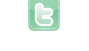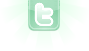This site requires JavaScript, please enable it in your browser!# Help

i Dont know whats wrong with this code can any one help me import greenfoot.*; // (World, Actor, GreenfootImage, and Greenfoot) public abstract class Mover extends Actor { private Vector movement = new Vector(); private int score; private double x = 0; private double y = 0; public Mover() { } /** * Create new mover initialised with given speed vector. */ public Mover(Vector speed) { movement = speed; } /** * Move forward one step. Direction and speed are dtermined by the * internal movement vector. Movement wraps around at the world edges. */ public void move() { x = x + movement.getX(); y = y + movement.getY(); if(x >= getWorld().getWidth()) { x = 0; } if(x < 0) { x = getWorld().getWidth() - 1; } if(y >= getWorld().getHeight()) { y = 0; } if(y < 0) { y = getWorld().getHeight() - 1; } setLocation(x, y); } public void setLocation(double x, double y) { this.x = x; this.y = y; super.setLocation((int) x, (int) y); } public void setLocation(int x, int y) { setLocation((double) x, (double) y); } /** * Increase the current speed with the given vector. */ public void increaseSpeed(Vector s) { movement.add(s); } /** * Return the current movement. */ public Vector getMovement() { return movement; } }
Stives123 wrote...
i Dont know whats wrong with this code can any one help me << Code Omitted >>
There is nothing wrong with the given Mover class code. However, there are two requirements to using it;; (1) you need to have a compatible Vector class; and (2) you must have a world that is not bounded.
You need to login to post a reply.